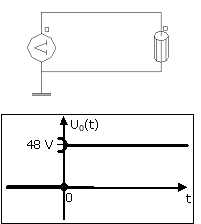# Initialization by static calculation: particular cases

## General case

The static initialization permits a computation close to reality (without numeric transient) in the cases where there are non-null sources of field at the initial time t = 0 s: magnets, currents (constant, three-phase...)

## Particular cases

There are a number of situations in which there are:

• non-null sources of field at the initial time t = 0 s (as described previously)
• and a change in the state of the circuit at t = 0 s
• Contactor (establishment of the voltage at t = 0 s)
• Closing switch
• Discharge of capacitor

These particular situations are presented in the blocks below.

## Contactor

Contactor with magnet; with establishment of the voltage at t = 0 s :

• initialization by static calculation allows to take into account the flux generated by the magnets
• to respect the "assumptions associated with the initialization by static calculation", the voltage should be set at t = 0 + epsilon

⇒ voltage is described with the function Valid or the function ValidLR as presented in the figure below

Finite element description: Circuit description:Coil around the core and the magnet is not represented

U(t) = U0*Valid(t, 1e-8,)

U(t) = U0*ValidLR(t,0, ,0,0)

## Switch

Device with magnet, and electrical circuit with a switch closes at t = 0 s :

• initialization by static calculation allows to take into account the flux generated by the magnets
• to respect the "assumptions associated with the initialization by static calculation", the switch must be closed at t = 0 + epsilon

⇒ see examples belowU0(t) = 48*ValidLR(t,0,,0,0) U0(t) = 5*cos(ω*t)*ValidLR(Time,0,,0,0)

## Capacitor

Device with magnet, and electrical circuit with discharge of a capacitor at t = 0s :

• initialization by static calculation allows to take into account the flux generated by the magnets
• to respect the "assumptions associated with the initialization by static calculation", you must:
• add a switch in series with the capacitor
• open the switch at t = 0 + epsilon